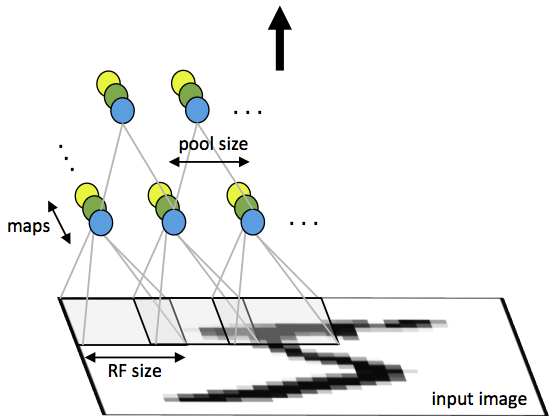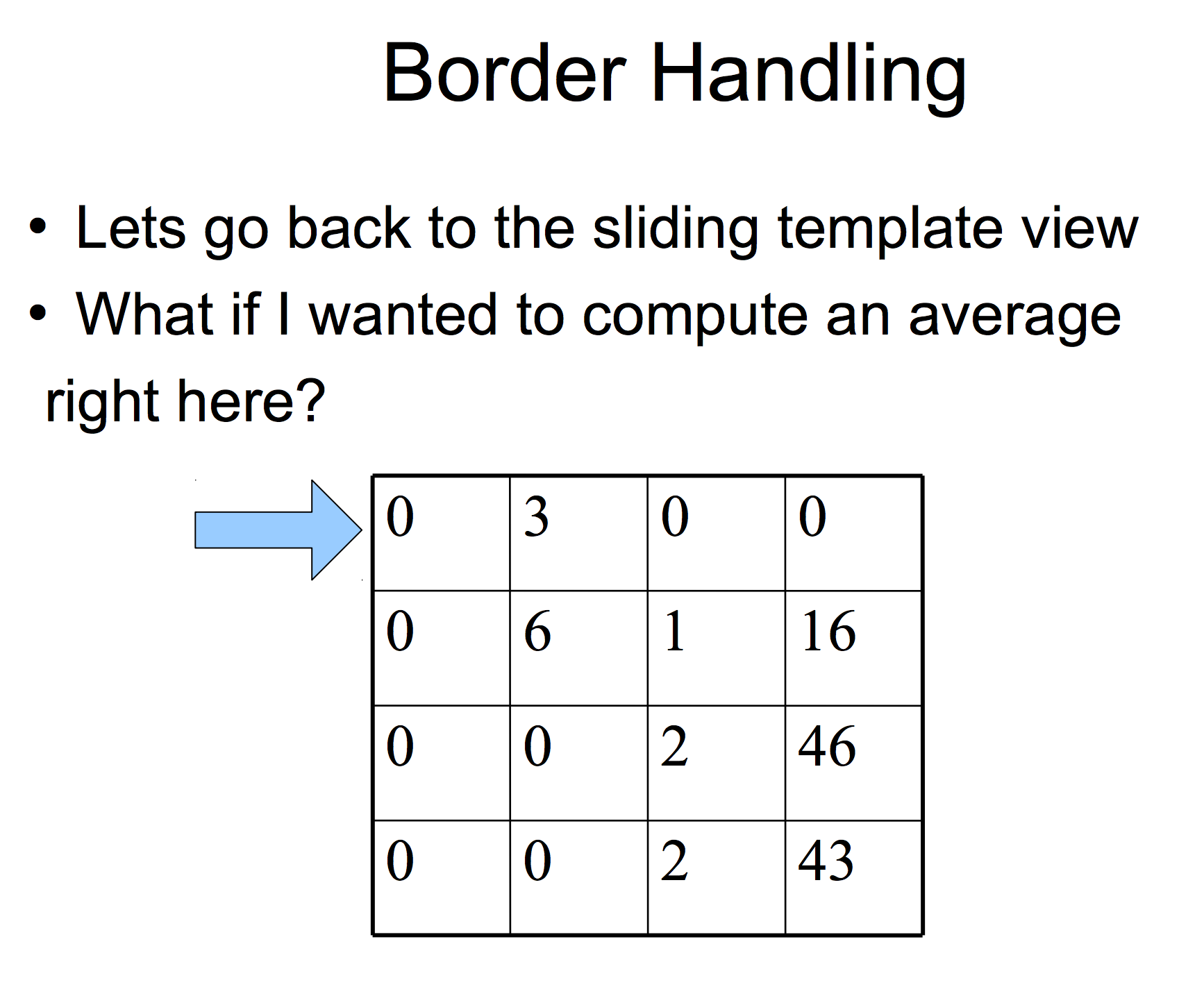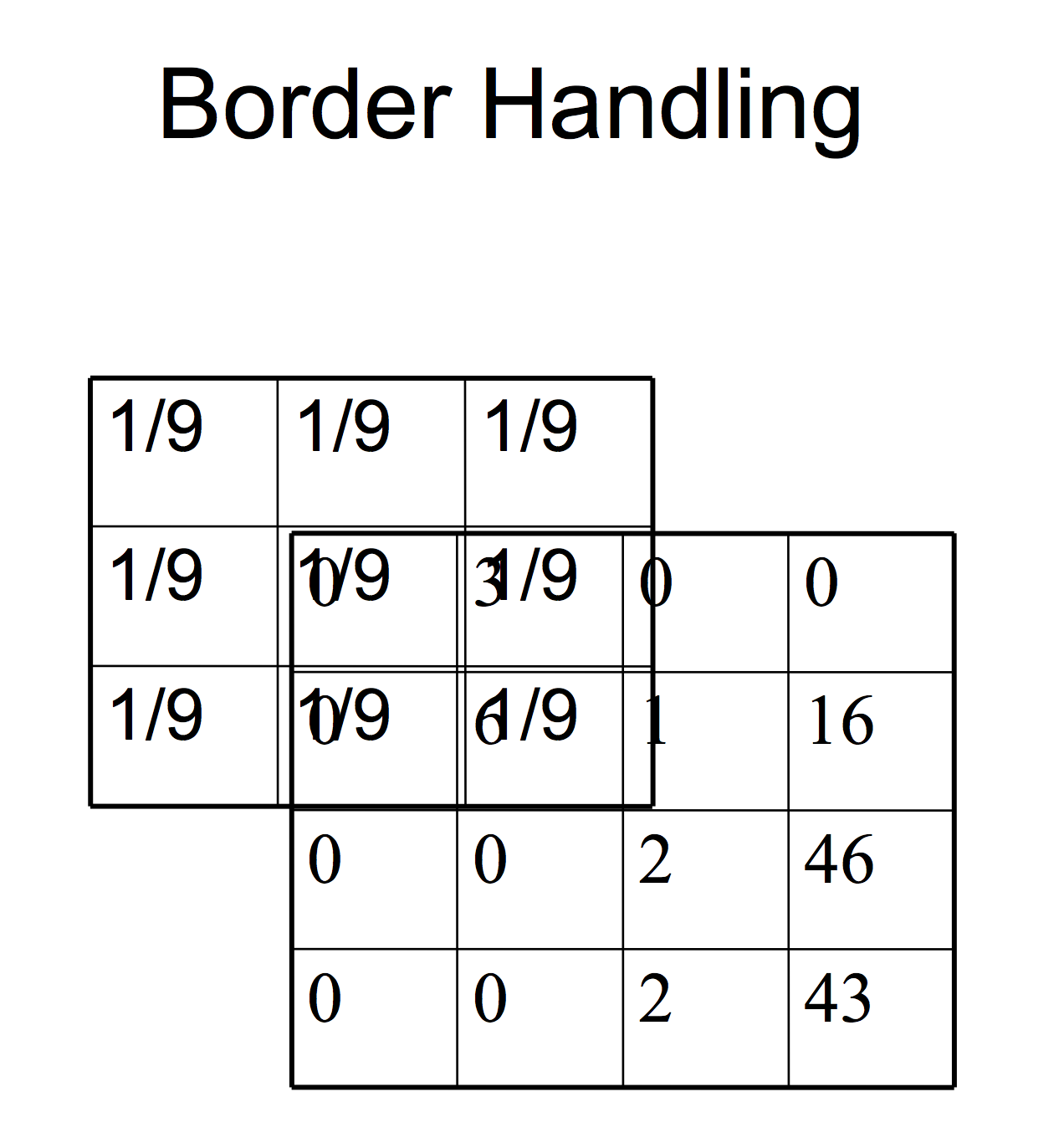# 随机梯度下降与卷积神经网络

## 随机梯度下降

$$\theta = \theta – \alpha \nabla_\theta E[J(\theta)]$$

$$\theta = \theta - \alpha \nabla_\theta J(\theta; x^{(i)},y^{(i)})$$

$SGD$ 中的学习率$\alpha$值比批梯度下降的学习率小很多，因为前者在更新过程中的方差更大。选择合适的学习率和学习率变更策略是相当困难的。一个实践有效的标准做法是在迭代开始时的一两个迭代中使用一个足够小的固定学习率，以提供了稳定的收敛性。然后随着收敛速度的下降，将学习率减半。一个更好的方法是每次迭代后在预留的一组数据上计算目标函数的值，当相邻两次迭代目标函数变化值小于某个较小的阈值时，才降低学习率。这往往会很好地收敛于一个局部最优值。另一个常用的策略是，学习率设为 $\frac{a}{b+t}$ ，其中 $t$ 是迭代回合数，变量 $a$ 和 $b$ 决定了初始时的学习率。还有更先进的方法，包括回溯线搜索寻找最优更新量。

### 动量

收敛速度特慢，特别是在第一次迭代之后。动量是推动目标函数值朝最优解的浅沟快速下降的一种方法。动量的更新过程如下：

\begin{align}v &= \gamma v+ \alpha \nabla_{\theta} J(\theta; x^{(i)},y^{(i)}) \\\theta &= \theta - v\end{align}

delta = momentum * prev_delta + gradient/batchsize

## 卷积神经网络

### 网络架构### 反向传播

$(x,y)$ 是标注训练数据。如果第 $l$ 层与第 $(l+1)$ 层是全连接的，那么第 $l$ 层的误差项为：

\begin{align} \delta^{(l)} = \left((W^{(l)})^T \delta^{(l+1)}\right) \bullet f'(z^{(l)}) \end{align}

\begin{align} \nabla_{W^{(l)}} J(W,b;x,y) &= \delta^{(l+1)} (a^{(l)})^T, \\ \nabla_{b^{(l)}} J(W,b;x,y) &= \delta^{(l+1)}.\end{align}

\begin{align} \delta_k^{(l)} = \text{upsample}\left((W_k^{(l)})^T \delta_k^{(l+1)}\right) \bullet f'(z_k^{(l)}) \end{align}\begin{align} \nabla_{W_k^{(l)}} J(W,b;x,y) &= \sum_{i=1}^m (a_i^{(l)}) \ast \text{rot90}(\delta_k^{(l+1)},2), \\ \nabla_{b_k^{(l)}} J(W,b;x,y) &= \sum_{a,b} (\delta_k^{(l+1)})_{a,b}. \end{align}

\begin{align} \nabla_{W^{(l)}} J(W,b;x,y) &= \delta^{(l+1)} (a^{(l)})^T, \\ \nabla_{b^{(l)}} J(W,b;x,y) &= \delta^{(l+1)}. \end{align}

### 评论 4

1. #3

楼主，你好！请问你这“文章侧边栏快速定位“和“文档中的公式“是使用的哪款插件啊

ishowcode7年前 (2017-04-13)回复
2. #2

写的真心不错，努力向你学习

ishowcode7年前 (2017-04-06)回复
3. #1

师兄，忽略博文情景问下吼，目前基于深度学习的日语机器翻译进展得如何了？/滑稽

sbd7年前 (2017-03-30)回复
• 我也不了解这个领域

hankcs7年前 (2017-03-31)回复